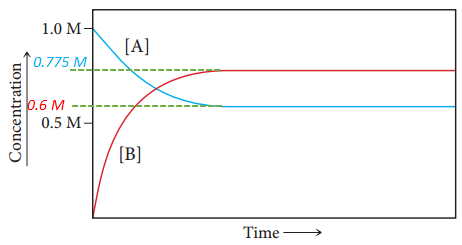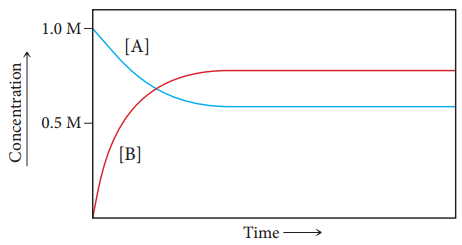# Problem: Consider the reaction: A(g) ⇌ 2B(g). The graph plots the concentrations of A and B as a function of time at a constant temperature.What is the equilibrium constant for this reaction at this temperature?

###### FREE Expert Solution

At equilibrium

• rate of forward reaction = rate of reverse reaction
• no observable change in concentration of products/reactants
• [A] = 0.6M
• [B] = 0.775 M• Forward: A(g) → 2B(g)
• Reverse: 2B(g) A(g)
• rate law = k[reactants]

$\overline{){{\mathbf{rate}}}_{{\mathbf{fwd}}}{\mathbf{=}}{{\mathbf{k}}}_{{\mathbf{fwd}}}\mathbf{\left[}\mathbf{A}\mathbf{\right]}}$     $\overline{){{\mathbf{rate}}}_{{\mathbf{rev}}}{\mathbf{=}}{{\mathbf{k}}}_{{\mathbf{rev}}}{\mathbf{\left[}\mathbf{B}\mathbf{\right]}}^{\mathbf{2}}}$

For a one step reaction, equilibrium constant K is:###### Problem Details
Consider the reaction: A(g) ⇌ 2B(g). The graph plots the concentrations of A and B as a function of time at a constant temperature.What is the equilibrium constant for this reaction at this temperature?